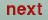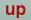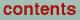Next: Category CHARGE Up: Category PLOT Previous: Tokens NDELR1NDELR2, and

### Token FORMAT= of cast integer (optional)

Selects the format of the output file. FORMAT=1, the format is appropriate for the GNUPLOT. =3, the format appropriate for DATA EXPLORER. =2, the format for 2D plots: x, y, f(x,y) and for 3D plots x, y, z, f(x,y,z), where x, y, and z are the x-, y-, and z-coordinate of the mesh point and f is the value of the function at this point. There is one mesh point per line. This format is appropriate for other plot packages.

O. Jepsen
Thu Oct 12 14:48:45 MESZ 2000